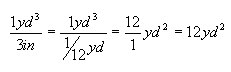From: Janet I need to spread landscape stone around my pool.  A landscaper said I need 16 yards.  What is the area of a cubic yard if I want the stone 3" deep? Thank you! Hi Janet. Cubic yards are a volume measure. You know the depth and want to know the area. Since volume = area X depth, then area = volume divided by depth. First, we need to convert so we are working with the same units. Three inches, if I recall correctly, is 1/12 of a yard. This means that the area (in square yards) that a cubic yard covers to a 3 inch depth is:So one (cubic) yard of stone covers 12 square yards around your pool to a depth of 3 inches. Remember though, that this doesn't include any room for wastage, error or settling - so you may need to add a bit of margin for that. Using this rate, you should be able to cover 16 X 12 = 192 square yards (1728 square feet) with 16 cubic yards of stone. Hope this helps! Sue